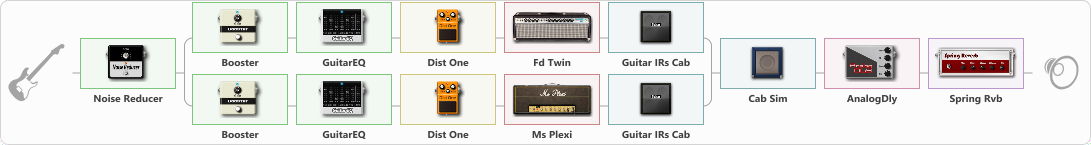# Avantasia

Discussion in 'ToneLib-GFX presets' started by truss van halen, Nov 10, 2020.

1. ### truss van halenWell-Known Member

Avantasia

Preset name: Avantasia

Effects chain:Effect: "Noise Reducer" (Dynamics / Filter), active - "yes"
{
"Sens" = 100
"Mode" = Hard
}

Effect: "Splitter" (Dynamics / Filter), active - "yes"
{
"A-Bypass" = Off
"A-Pan" = 0
"A-Level" = 55
"B-Bypass" = Off
"B-Pan" = 0
"B-Level" = 55

'A' branch:
{

Effect: "Booster" (Dynamics / Filter), active - "yes"
{
"Gain" = 25
}

Effect: "GuitarEQ" (Dynamics / Filter), active - "yes"
{
"160 Hz" = 0
"400 Hz" = 0
"800 Hz" = 0
"1.6 kHz" = 0
"3.2 kHz" = 0
"6.4 kHz" = 0
"12 kHz" = 0
"Level (dB)" = 0
}

Effect: "Dist One" (Overdrive / Distortion), active - "yes"
{
"Drive" = 50
"Tone" = 73
"Level" = 69
}

Effect: "Fd Twin" (Amp simulators), active - "yes"
{
"Gain" = 49
"Bass" = 36
"Middle" = 57
"Treble" = 74
"Presence" = 67
"Master" = 68
"Level (dB)" = 7
}

Effect: "Guitar IRs Cab" (Cabinets), active - "yes"
{
"Model" = Fender Deluxe Rev (1x12")
"Mic Position" = Middle
"Mic Distance" = Near
"Low Cut (Hz)" = 60
"Hi Cut (kHz)" = 20.0
"Mix" = 100
"Level (dB)" = 0
}
}
'B' branch:
{

Effect: "Booster" (Dynamics / Filter), active - "yes"
{
"Gain" = 74
}

Effect: "GuitarEQ" (Dynamics / Filter), active - "yes"
{
"160 Hz" = 0
"400 Hz" = 3
"800 Hz" = 6
"1.6 kHz" = 0
"3.2 kHz" = 0
"6.4 kHz" = 0
"12 kHz" = 0
"Level (dB)" = 0
}

Effect: "Dist One" (Overdrive / Distortion), active - "yes"
{
"Drive" = 50
"Tone" = 73
"Level" = 69
}

Effect: "Ms Plexi" (Amp simulators), active - "yes"
{
"Gain" = 71
"Bass" = 50
"Middle" = 50
"Treble" = 50
"Presence" = 50
"Master" = 50
"Level (dB)" = 0
}

Effect: "Guitar IRs Cab" (Cabinets), active - "yes"
{
"Model" = Fender Deluxe Rev (1x12")
"Mic Position" = Middle
"Mic Distance" = Near
"Low Cut (Hz)" = 60
"Hi Cut (kHz)" = 20.0
"Mix" = 100
"Level (dB)" = 0
}
}
}

Effect: "Cab Sim" (Cabinets), active - "yes"
{
"Model" = 2x12" Blackface
"Level (dB)" = 0
}

Effect: "AnalogDly" (Delay), active - "yes"
{
"Time" = 652
"Feedback" = 17
"Tone" = 65
"Mix" = 65
}

Effect: "Spring Rvb" (Reverberation), active - "yes"
{
"Time" = 6.7
"PreDelay" = 53
"LoDamp" = 70
"HiDamp" = 35
"Mix" = 27
}

Note: You will need to download and install the ToneLib-GFX software to use the preset.

File size:
1.3 KB
Views:
2,609